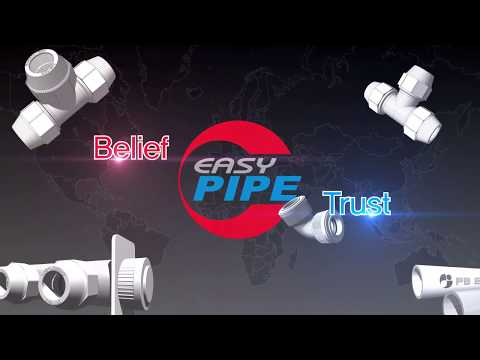# PB PIPE of SAEROMTECH

Product

•PB PIPE ( COLL&HOT WATERSUPPLY / HEATING / KS M 3363 : 2009)

< Thermal conductivity >
PB pipes have the advantage of less thermal conductivity and less heat loss than metal pipes.
It is therefore more efficient than metal pipes. However, if you want to minimize long-distance piping or heat loss, use a...

## Description of the Product

PB PIPE ( COLL&HOT WATERSUPPLY / HEATING / KS M 3363 : 2009)

< Thermal conductivity >
PB pipes have the advantage of less thermal conductivity and less heat loss than metal pipes.
It is therefore more efficient than metal pipes. However, if you want to minimize long-distance piping or heat loss, use a thermal insulation material.

< Linear expansion coefficient >
Since the coefficient of linear expansion of PB pipe is larger than the coefficient of linear expansion of metal pipe, the coefficient of thermal expansion is relatively large.
Therefore, pay attention to the fixing method. However, the tensile modulus is so low that it becomes a fixed pipe without the need to use an expansion joint.

## Price of the Product

Price not indicated